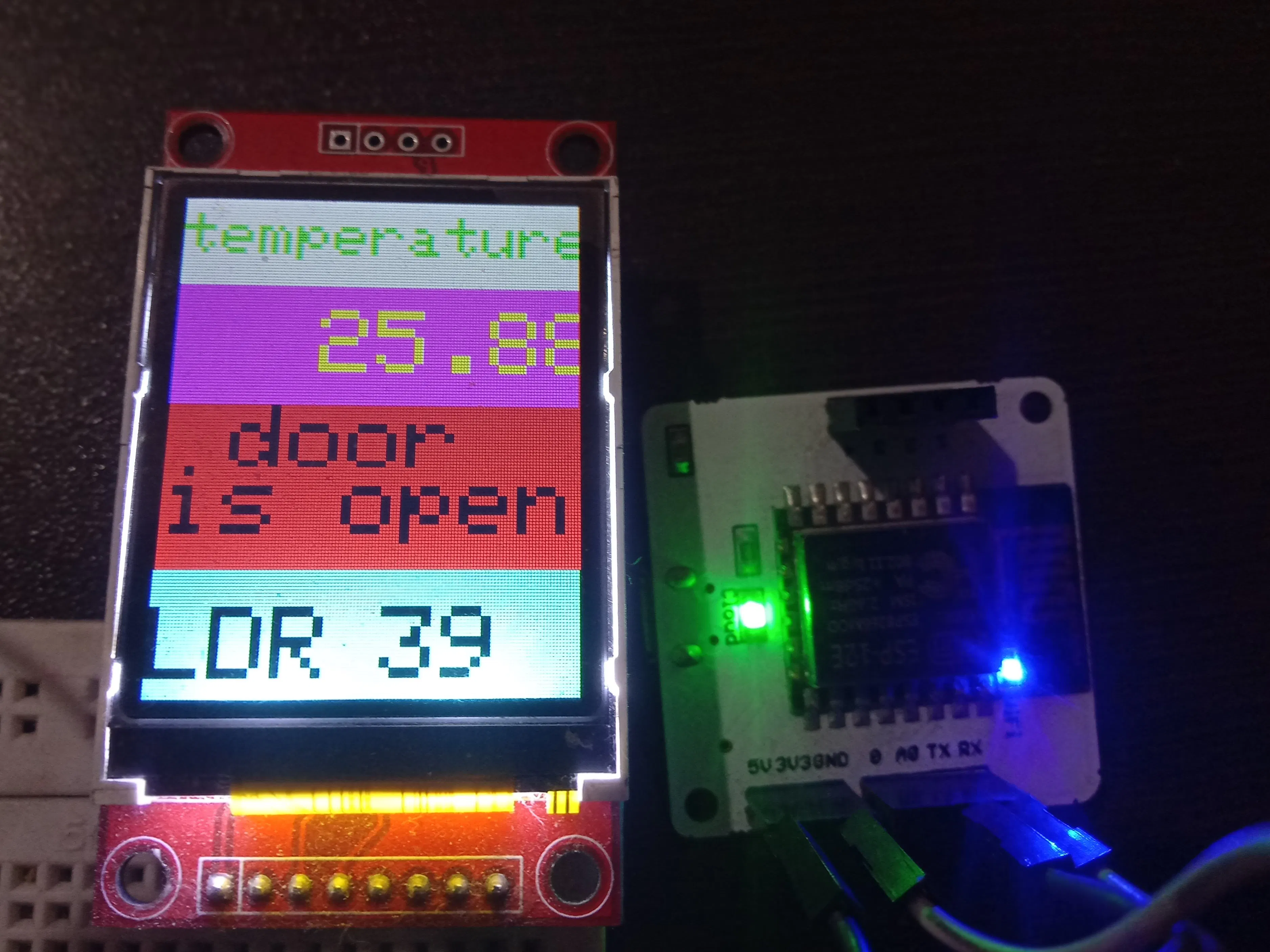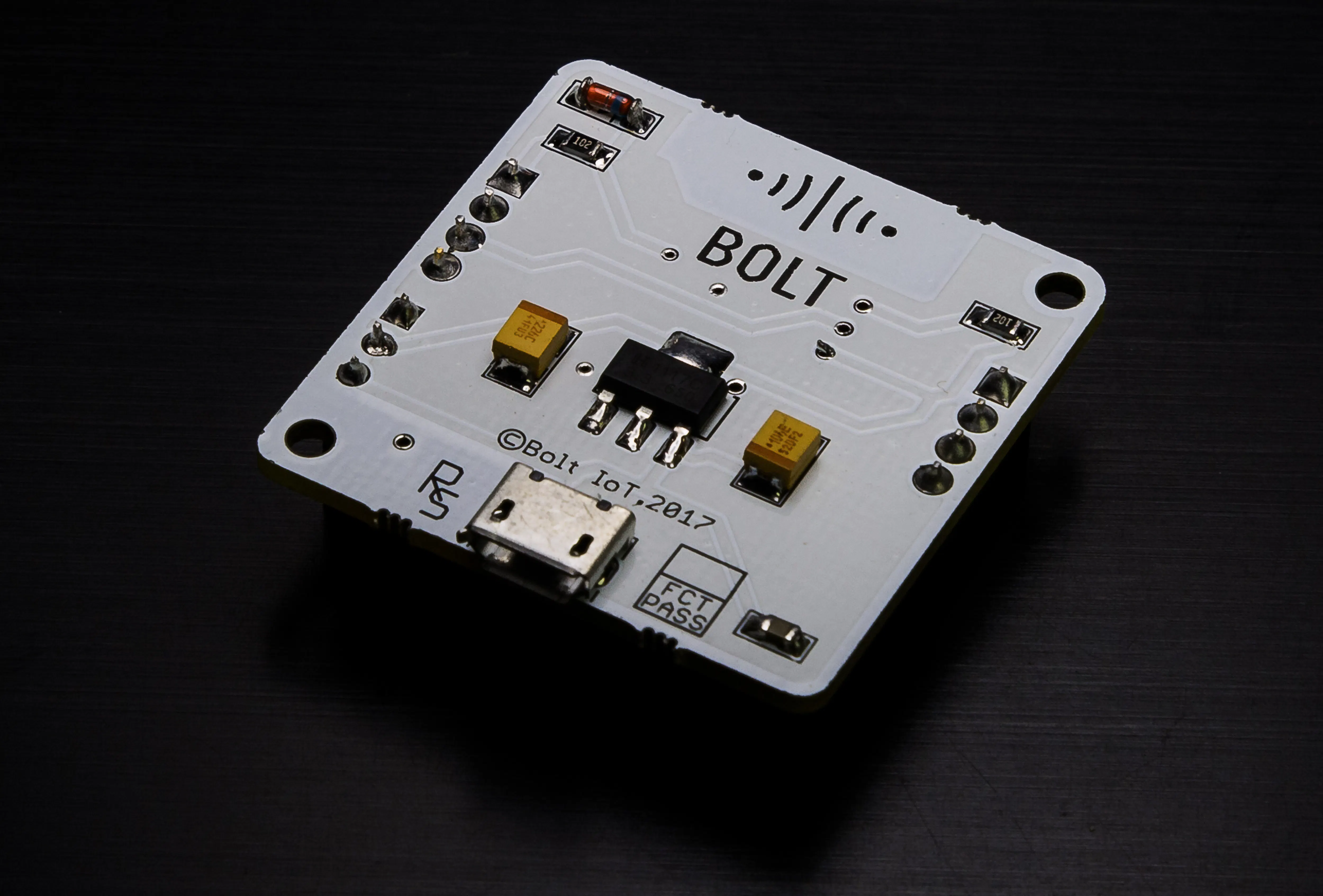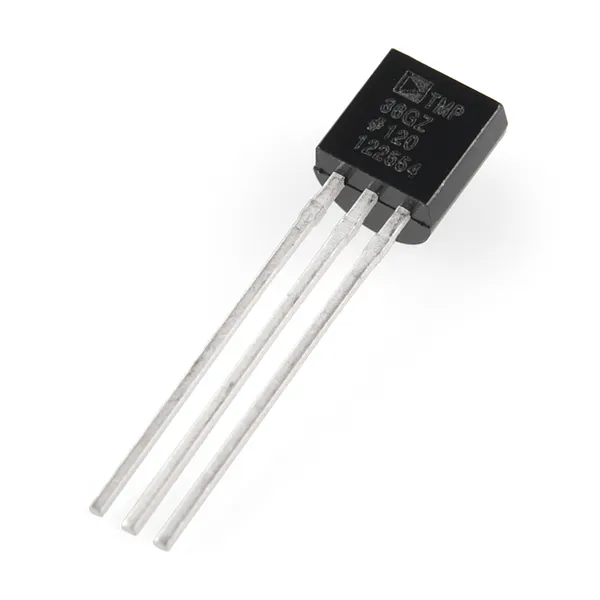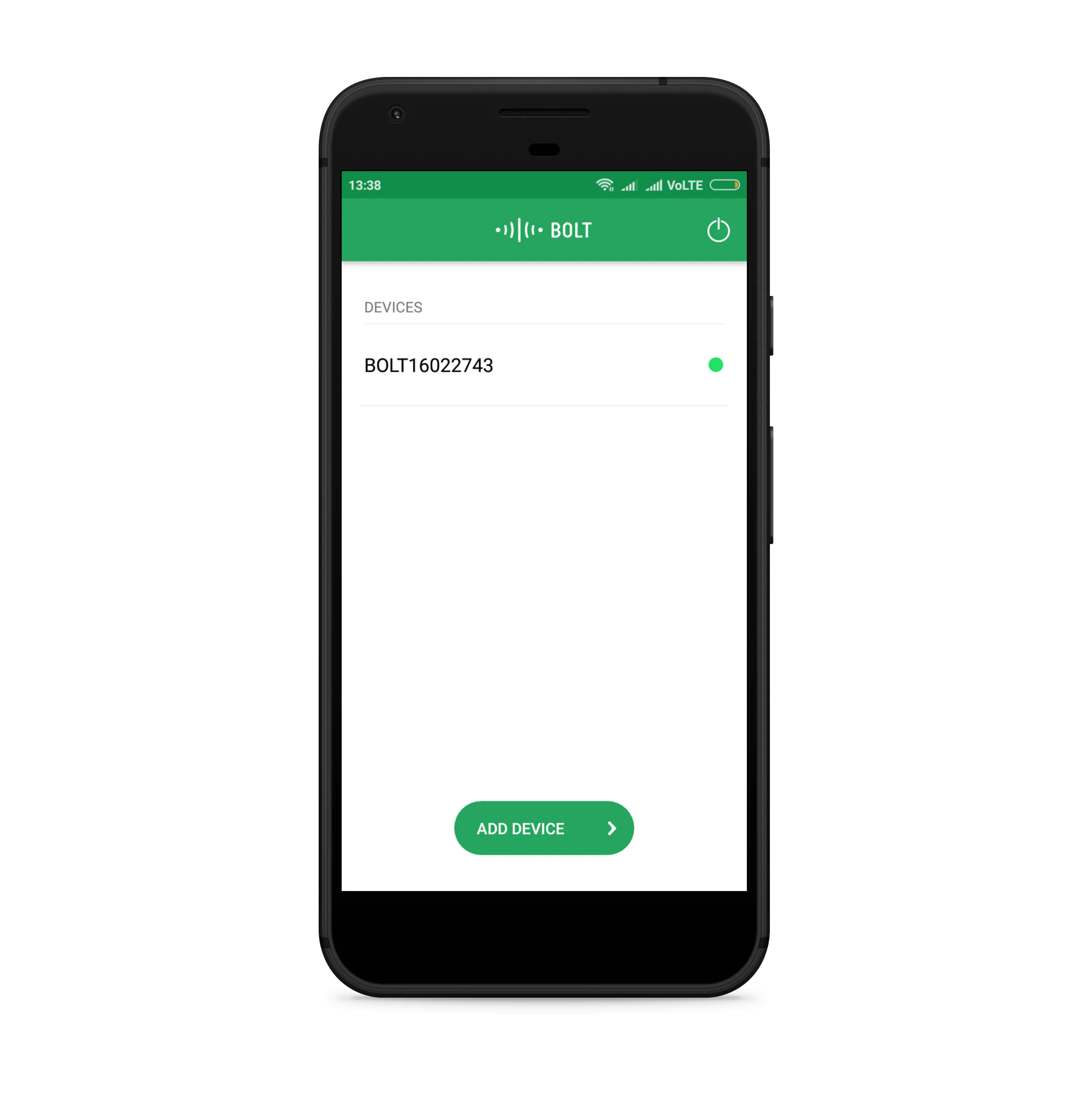# Anomaly Detection & Temperature Monitoring Using Bolt IoT

Use Bolt IoT kit and Arduino Uno to make your own anomaly detection & temperature monitoring device in both modes, i. e online and offline.## Things used in this project

### Hardware componentsBolt IoT Bolt WiFi Module
×1Arduino UNO
×1Temperature Sensor
×1
 LDR
×1SparkFun Pushbutton switch 12mm
×1
 TFT 1.8 display
×1Jumper wires (generic)
×1Resistor 10k ohm
×2
 Power Bank
×1

### Software apps and online servicesBolt IoT Bolt CloudBolt IoT Android AppArduino IDE
 DigitaloceanTwilio SMS Messaging API
 @mailgum

## Code

### main code for Arduino Uno

C/C++
this code for Arduino Uno
```#include <Adafruit_GFX.h>  // Include core graphics library
#include <BoltIoT-Arduino-Helper.h>//Include boltiot library

// Declare pins for the display:
#define TFT_CS     10
#define TFT_RST    8
#define TFT_DC     9
// The rest of the pins are pre-selected as the default hardware SPI for Arduino Uno (SCK = 13 and SDA = 11)

// Create display:
//variables
int inPin = 2;  //for push button
int push_button = 0;
int lm35_sensor_value;
int intensity_of_light;
float cel ;

String getAnalogData(String *data){
String retval;
retval=cel;
return retval;
}

void setup()  // Start of setup
{

Serial.begin(9600);
boltiot.begin(6,7);
boltiot.setCommandString("GetAnalogData",getAnalogData);

// Display setup:
tft.setTextWrap(false);
// Use this initializer if you're using a 1.8" TFT
tft.initR(INITR_BLACKTAB);  // Initialize a ST7735S chip, black tab
tft.fillScreen(ST7735_BLACK);  // Fill screen with black

//tft.setRotation(0);  // Set orientation of the display. Values are from 0 to 3. If not declared, orientation would be 0,

// Draw rectangle:
tft.fillRect(0, 0, 129, 30,ST7735_WHITE);
tft.fillRect(0,30,129,40,ST7735_MAGENTA);
tft.fillRect(0,115,129,45,ST7735_CYAN);
// tft.drawRect(0, 30, 129, 40, ST7735_WHITE);

// Write to the display the text "Hello":

tft.setCursor(0, 5);  // Set position (x,y)
tft.setTextColor( ST7735_GREEN,ST7735_WHITE); // Set color of text. First is the color of text and after is color of background
tft.setTextSize(2);  // Set text size. Goes from 0 (the smallest) to 20 (very big)
tft.println("temperature");  // Print a text or value

}  // End of setup

void loop()  // Start of loop
{

float mv = ( lm35_sensor_value/1024.0)*5000;
cel = mv/10;
// float farh = (cel*9)/5 + 32;

Serial.print("TEMPRATURE = ");
Serial.print(cel);
Serial.print("*C");
Serial.println();
//boltiot.processPushDataCommand(cel);

boltiot.handleCommand();

if (push_button == HIGH && intensity_of_light < 20 ) {   // door closed

tft.fillRect(0,70,129,45,ST7735_GREEN);
tft.setCursor(0, 70);  // Set position (x,y)
tft.setTextColor(ST7735_BLACK, ST7735_GREEN);  // Set color of text. First is the color of text and after is color of background
tft.setTextSize(3);  // Set text size. Goes from 0 (the smallest) to 20 (very big)
tft.println("door is");
tft.setCursor(0, 90);  // Set position (x,y)
tft.setTextColor(ST7735_BLACK, ST7735_GREEN);  // Set color of text. First is the color of text and after is color of background
tft.setTextSize(3);  // Set text size. Goes from 0 (the smallest) to 20 (very big)
tft.println("closed");

} else {         // door open
tft.fillRect(0,70,129,52,ST7735_RED);
tft.setCursor(19, 70);  // Set position (x,y)
tft.setTextColor(ST7735_BLACK, ST7735_RED);  // Set color of text. First is the color of text and after is color of background
tft.setTextSize(3);  // Set text size. Goes from 0 (the smallest) to 20 (very big)
tft.println("door");
tft.setCursor(0, 90);  // Set position (x,y)
tft.setTextColor(ST7735_BLACK, ST7735_RED);  // Set color of text. First is the color of text and after is color of background
tft.setTextSize(3);  // Set text size. Goes from 0 (the smallest) to 20 (very big)
tft.println("is open");
}

tft.setCursor(0, 133);  // Set position (x,y)
tft.setTextColor(ST7735_BLACK,  ST7735_CYAN );  // Set color of text. First is the color of text and after is color of background
tft.setTextSize(3);
tft.println("LDR ");
tft.setCursor(68, 133);
tft.println(intensity_of_light);

tft.setCursor(45, 38);  // Set position (x,y)
tft.setTextColor(ST7735_YELLOW,ST7735_MAGENTA);  // Set color of text. First is the color of text and after is color of background
tft.setTextSize(3);  // Set text size. Goes from 0 (the smallest) to 20 (very big)
tft.println(cel);

delay(1000);

}  // End of loop
```

### Code for data fetching from boltiot and checking threshold and anomaly detection

Python
Run this code on your server
```import conf, email_conf, json, time, math, statistics
from boltiot import Sms, Email, Bolt

def compute_bounds(history_data,frame_size,factor):
if len(history_data)<frame_size :
return None

if len(history_data)>frame_size :
del history_data[0:len(history_data)-frame_size]
Mn=statistics.mean(history_data)
Variance=0
for data in history_data :
Variance += math.pow((data-Mn),2)
Zn = factor * math.sqrt(Variance / frame_size)
High_bound = history_data[frame_size-1]+Zn
Low_Bound = history_data[frame_size-1]-Zn
return [High_bound,Low_Bound]

minimum_limit = 4
maximum_limit = 7

mybolt = Bolt(email_conf.API_KEY, email_conf.DEVICE_ID)
mailer = Email(email_conf.MAILGUN_API_KEY, email_conf.SANDBOX_URL, email_conf.SENDER_EMAIL, email_conf.RECIPIENT_EMAIL)
sms = Sms(conf.SSID, conf.AUTH_TOKEN, conf.TO_NUMBER, conf.FROM_NUMBER)

history_data=[]

while True:

if data['success'] != '1':
print("There was an error while retriving the data.")
print("This is the error:"+data['value'])
time.sleep(10)
continue

print ("The sensor value is "+(data['value']))
sensor_value=0
try:
sensor_value = int(data['value'])

except Exception as e:
print("There was an error while parsing the response: ",e)
continue

bound = compute_bounds(history_data,email_conf.FRAME_SIZE,email_conf.MUL_FACTOR)
if not bound:
required_data_count=email_conf.FRAME_SIZE-len(history_data)
print("Not enough data to compute Z-score. Need ",required_data_count," more data points")
history_data.append(int(data['value']))
time.sleep(10)
continue

try:
if sensor_value > maximum_limit or sensor_value < minimum_limit:
response = mailer.send_email("Alert", "The Current temperature is beyond the threshold ")
if sensor_value > bound :
print ("The temperature increased suddenly. Sending Sms.")
response = sms.send_sms("Someone opened the chamber")
print("This is the response ",(response))
elif sensor_value < bound:
print ("The temperature decreased suddenly. Sending an email.")
response = mailer.send_email("Someone opened the chamber")
print("This is the response ",response)
history_data.append(sensor_value);
except Exception as e:
print ("Error",e)
time.sleep(10)
```

### conf.py

Python
include this file (import conf) in main code for sms sending
```SSID = 'You can find SSID in your Twilio Dashboard'
AUTH_TOKEN = 'You can find  on your Twilio Dashboard'
FROM_NUMBER = 'This is the no. generated by Twilio. You can find this on your Twilio Dashboard'
TO_NUMBER = 'This is your number. Make sure you are adding +91 in beginning'
API_KEY = 'This is your Bolt Cloud account API key'
DEVICE_ID = 'This is the ID of your Bolt device'
FRAME_SIZE = 10
MUL_FACTOR = 6
```

### email_conf.py

Python
```MAILGUN_API_KEY = 'This is the private API key which you can find on your Mailgun Dashboard'
SANDBOX_URL= 'You can find this on your Mailgun Dashboard'
SENDER_EMAIL = 'This would be test@your SANDBOX_URL'
RECIPIENT_EMAIL = 'Enter your Email ID Here'
API_KEY = 'This is your Bolt Cloud accout API key'
DEVICE_ID = 'This is the ID of your Bolt device'
FRAME_SIZE = 10
MUL_FACTOR = 6
```

## Credits

### ashish anand

1 project • 26 followers
Love to find solutions and learn new things